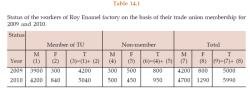Courses

# Test: Statistical Description Of Data - 1

## 40 Questions MCQ Test Quantitative Aptitude for CA CPT | Test: Statistical Description Of Data - 1

Description
This mock test of Test: Statistical Description Of Data - 1 for CA Foundation helps you for every CA Foundation entrance exam. This contains 40 Multiple Choice Questions for CA Foundation Test: Statistical Description Of Data - 1 (mcq) to study with solutions a complete question bank. The solved questions answers in this Test: Statistical Description Of Data - 1 quiz give you a good mix of easy questions and tough questions. CA Foundation students definitely take this Test: Statistical Description Of Data - 1 exercise for a better result in the exam. You can find other Test: Statistical Description Of Data - 1 extra questions, long questions & short questions for CA Foundation on EduRev as well by searching above.
QUESTION: 1

Solution:
QUESTION: 2

Solution:
QUESTION: 3

### Statistics is applied in

Solution:
QUESTION: 4

Statistics is concerned with

Solution:
QUESTION: 5

An attribute is

Solution:
QUESTION: 6

Annual income of a person is

Solution:
QUESTION: 7

Marks of a student is an example of

Solution:
QUESTION: 8

Nationality of a student is

Solution:
QUESTION: 9

Drinking habit of a person is

Solution:
QUESTION: 10

Age of a person is

Solution:
QUESTION: 11

Data collected on religion from the census reports are

Solution:
QUESTION: 12

The data collected on the height of a group of students after recording their heights with a measuring tape are

Solution:
QUESTION: 13

The primary data are collected by

Solution:
QUESTION: 14

The quickest method to collect primary data is

Solution:
QUESTION: 15

The best method to collect data, in case of a natural calamity, is

Solution:

Yes, personal interview is one of the best option. As it actually gives the information of calamity and also of what happens in a clear way and it enables us to know the actual experience of a person

QUESTION: 16

In case of a rail accident, the appropriate method of data collection is by

Solution:
QUESTION: 17

Which method of data collection covers the widest area?

Solution:

Option (b) mailed Questionarie method is right answer.

Explanation:-

(a) Telephone can be used to near and far of places, there is a chance that other person  at the far end may not hear properly so this fails.

( b) Mails are the best, the reason at anytime in a day one can go through and send replies comfortably  . So this is the correct answer.
( c) Direct interview method some times doesn't suit.

QUESTION: 18

The amount of non-responses is maximum in

Solution:
QUESTION: 19

Some important sources of secondary data are

Solution:
QUESTION: 20

Internal consistency of the collected data can be checked when

Solution:
QUESTION: 21

The accuracy and consistency of data can be verified by

Solution:
QUESTION: 22

The mode of presentation of data are

Solution:
QUESTION: 23

The best method of presentation of data is

Solution:
QUESTION: 24

The most attractive method of data presentation is

Solution:
QUESTION: 25

For tabulation, ‘caption’ is

Solution:
QUESTION: 26

‘Stub’ of a table is the

Solution:
QUESTION: 27

The entire upper part of a table is known as

Solution:
QUESTION: 28

The unit of measurement in tabulation is shown in

Solution:
QUESTION: 29

In tabulation source of the data, if any, is shown in the

Solution:
QUESTION: 30

Which of the following statements is untrue for tabulation?

Solution:
QUESTION: 31

Hidden trend, if any, in the data can be noticed in

Solution:
QUESTION: 32

Diagrammatic representation of data is done by

Solution:
QUESTION: 33

The most accurate mode of data presentation is

Solution:
QUESTION: 34

The chart that uses logarithm of the variable is known as

Solution:
QUESTION: 35

Multiple line chart is applied for

Solution:
QUESTION: 36

Multiple axis line chart is considered when

Solution:

multi-axis line chart is an interactive line chart that plots data using multiple axes. This allows plotting data sets having different units and scale ranges. Data values are plotted as data points that are connected by line segments. Multiple y-axes are rendered along the left and right side of the chart.

QUESTION: 37

Horizontal bar diagram is used for

Solution:
QUESTION: 38

Vertical bar diagram is applicable when

Solution:
QUESTION: 39

Divided bar chart is considered for

Solution:
QUESTION: 40

In order to compare two or more related series, we consider

Solution:

Track your progress, build streaks, highlight & save important lessons and more!

### Similar Content### Related tests